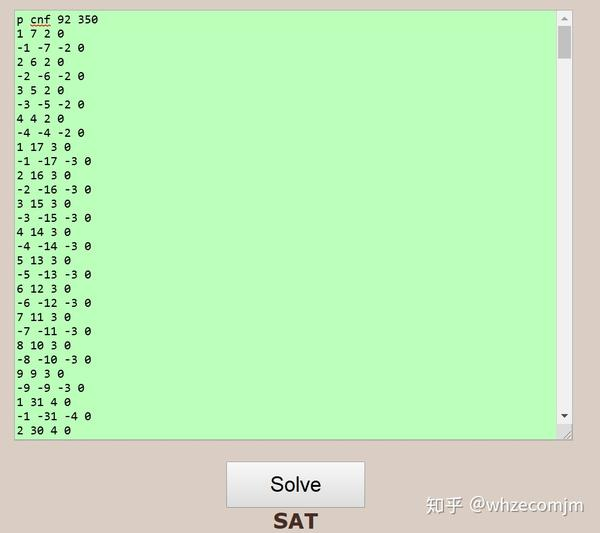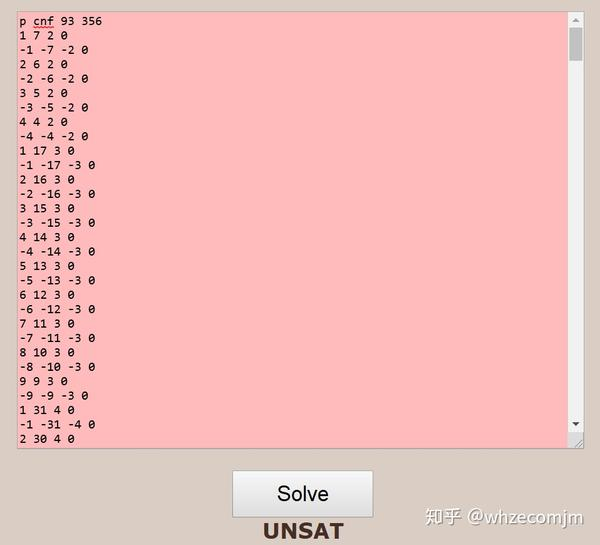# 方程的染色问题

Definition 1. An equation $f(x,y,z,\dots)=0$ is partition regular (or simply regular) if, and only if, for any finite partition of $\mathbb{N}$ into $C_1, C_1,\dots, C_r$, there exists some $C_i$ containing a non-trivial solution to the equation.

Schur 最早研究了分割正规的Schur方程，也就是最简单的线性齐次方程 $x+y=z$。Schur定理表明，对自然数的任意有限染色，上述方程都有单色解。为了更好的理解，我们考虑二染色，用反证法可以证明在二染色情况下，总存在单色解。

Definition 2. Given an equation or a system of equations $\mathcal{L}$ and finite $r$ colors, the Rado number $R(\mathcal{L},r)$ is the least number such that any $r$-coloring of ${1,2,\dots,R(\mathcal{L},r)}$ must contain a monochromatic solution to $\mathcal{ L}$.

Theorem 3 (Rado’s Theorem ). A linear homogeneous equation $a_1 x_1+\cdots+a_nx_n=0$ is partition regular on $\mathbb{N}$ if and only if there exists a nonempty set $J\subseteq{1,\dots,n}$ such that $\sum_{j\in J}a_j=0$.

Definition 4. The degree of regularity of an equation or a system of equations $\mathcal{L}$ is the largest integer $r\geq 0$, if any, such that $\mathcal{L}$ is $r$-regular. This number is denoted by $\mathrm{dor}(\mathcal{L})$. In particular, if $\mathrm{dor}(\mathcal{L})=\infty$, then $\mathcal{L}$ is regular.

$$R(x+y=2z^2,2)=93.$$ 下面附上生成 cpf 的python 代码（几年没写过代码）和结果：

n=94; # then 93
# get the number of clauses for any n<100.
def clauses(n,k=0):
for j in range(2,10):
for i in range(1,j**2+1):
if i <n and 2*j**2-i<n:
k=k+2
return k

# print the head of the cpf file.
print ("p cnf",n-1,clauses(n))

# print contents of the cpf file
for j in range(2,10): # for n<100, 1 < z <10
for i in range(1,j**2+1): # get rid of symmetric solutions of x and y's
if i <n and 2*j**2-i<n: # all solutions with x, y less than n
print (i, 2*j**2-i, j, 0) # the condition of no blue monochromatic
print (-i, -2*j**2+i, -j, 0) # the condition of no red monochromaticReferences.

 R. Rado, Studien zur Kombinatorik, Math. Z. 36 (1933), 242–280.
 S. W. Golomb and L. D. Baumert. “Backtrack programming.” Journal of the ACM (JACM) 12.4 (1965): 516-524.
 M. Heule, Schur number five. Thirty-Second AAAI Conference on Artificial Intelligence. 2018.
 M. Heule, O. Kullmann, and V. Marek, Solving and verifying the boolean Pythagorean triples problem via cube-and-conquer. International Conference on Theory and Applications of Satisfiability Testing. Springer, Cham, 2016.
 M. Nasso and L. Baglini. Ramsey properties of nonlinear Diophantine equations. Advances in Mathematics 324 (2018): 84-117.
 B. Green and S. Lindqvist. Monochromatic Solutions to x+y=z2. Canadian Journal of Mathematics 71.3 (2019): 579-605.##### Wenchao Zhang
###### PhD Student in Mathematics

My research interests include ring and algebra, algebraic geometry and some combinatorics.